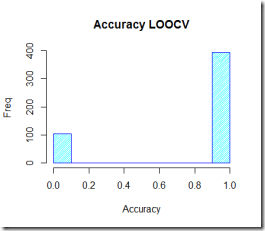Want to share your content on R-bloggers? click here if you have a blog, or here if you don't.

Now that we fitted the classifier and run some preliminary tests, in order to get a grasp at how our model is doing when predicting creditability we need to run some cross validation methods.
Cross validation is a model evaluation method that does not use conventional fitting measures (such as R^2 of linear regression) when trying to evaluate the model. Cross validation is focused on the predictive ability of the model. The process it follows is the following: the dataset is splitted in a training and testing set, the model is trained on the testing set and tested on the test set.

Note that running this process one time gives you a somewhat unreliable estimate of the performance of your model since this estimate usually has a non-neglectable variance.

In order to get a better estimate of model performance I am going to use a variant of the famous k-fold cross validation. I am going to split the dataset into a training set (95% of the data) and a test set (5% of the data) randomly for k different times and measure accuracy, false positive rate and false negative rate. After this I am going to run a double check using leave-one-out cross validation (LOOCV). LOOCV is a K-fold cross validation taken to its extreme: the test set is 1 observation while the training set is composed by all the remaining observations. Note that in LOOCV K = number of observations in the dataset.
While there are some built-in functions in R that let you run cross validation, often more efficiently than implementing the process by yourself, this time I prefer coding my own because I would like to have a better understanding of what the algorithm is doing and how it is doing it. Although we may be paying a little more in terms of efficiency, by building our own “cross validator” we can personalize and tweak most of the parameters and therefore achieve a better process customization.

### Cross validation (K-fold variant)

Let’s run (customized) cross validation first, then LOOCV

By averaging the accuracy score we get an average of 0.78856, not bad at all! Then, we need to plot the histogram and the boxplot of the accuracy to get a better ideaBy looking at the plots you can see that measuring performance on a single train-test splitting may well be deceiving! On average our classifier is doing quite good: all the accuracy scores are greater than 0.5 and 75% of the accuracy are greater than 0.7. That’s at least a good start.

### Leave one out cross validation (LOOCV)

Let’s try LOOCV now! Cross validation to its extreme: our classifier is tested on one observation only and trained on the remaining ones. Note that here the number of iterations is limited by the number of samples (the number of rows in the dataset).

By running the script I get 0.79 average accuracy score. This is great and seems to be consistent with the score we got before using the previous cross validation method. We can plot the results although the plot does not necessarily add any significant insight.As a comparison, we can try to run the cross validation function in R in the boot package:

# CV using boot

library(boot)

# Cost function for a binary classifier suggested by boot package

# (otherwise MSE is the default)

cost <- function(r, pi = 0) mean(abs(r-pi) > 0.5)

# LOOCV (accuracy)

1-cv.glm(dat,model,cost=cost)$delta # OUTPUT:  0.79 # K-fold CV K=10 (accuracy) 1-cv.glm(dat,model,K=10,cost=cost)$delta

# OUTPUT:  0.79

It seems that everything we did was fine and consistent with R built-in functions. Good!